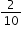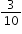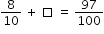# MAFS.4.NF.3.5Archived Standard

Express a fraction with denominator 10 as an equivalent fraction with denominator 100, and use this technique to add two fractions with respective denominators 10 and 100. For example, express 3/10 as 30/100, and add 3/10 + 4/100 = 34/100.

General Information
Subject Area: Mathematics
Domain-Subdomain: Number and Operations - Fractions
Cluster: Level 1: Recall
Cluster: Understand decimal notation for fractions, and compare decimal fractions. (Major Cluster) -

Clusters should not be sorted from Major to Supporting and then taught in that order. To do so would strip the coherence of the mathematical ideas and miss the opportunity to enhance the major work of the grade with the supporting clusters.

Date of Last Rating: 02/14
Status: State Board Approved - Archived
Assessed: Yes
Test Item Specifications

• Assessment Limits :
Denominators must be either 10 or 100. Decimal notation may not be assessed at this standard.
• Calculator :

No

• Context :

Allowable

Sample Test Items (4)
• Test Item #: Sample Item 1
• Question:

Create a fraction with a denominator of 100 that is equivalent to.

• Difficulty: N/A
• Type: EE: Equation Editor

• Test Item #: Sample Item 2
• Question:

Which fraction is equivalent to?

• Difficulty: N/A
• Type: MC: Multiple Choice

• Test Item #: Sample Item 3
• Question:

An equation is shown.What is the missing fraction?

• Difficulty: N/A
• Type: EE: Equation Editor

• Test Item #: Sample Item 4
• Question:

Melvin mows a lawn. The fraction of the lawn that Melvin has mowed so far is represented by the shaded model shown.Melvin will mowmore of the lawn before he takes his first break.

What fraction of the lawn will Melvin have mowed when he takes his first break?

• Difficulty: N/A
• Type: EE: Equation Editor

## Related Courses

This benchmark is part of these courses.
5012060: Mathematics - Grade Four (Specifically in versions: 2014 - 2015, 2015 - 2022, 2022 and beyond (current))
7712050: Access Mathematics Grade 4 (Specifically in versions: 2014 - 2015, 2015 - 2018, 2018 - 2022, 2022 and beyond (current))
5020110: STEM Lab Grade 4 (Specifically in versions: 2016 - 2022, 2022 and beyond (current))
5012065: Grade 4 Accelerated Mathematics (Specifically in versions: 2019 - 2022, 2022 and beyond (current))
5012015: Foundational Skills in Mathematics 3-5 (Specifically in versions: 2019 - 2022, 2022 and beyond (current))

## Related Access Points

Alternate version of this benchmark for students with significant cognitive disabilities.

## Related Resources

Vetted resources educators can use to teach the concepts and skills in this benchmark.

## Formative Assessments

Tenths and Hundredths:

Students are asked if an equation involving the sum of two fractions is true or false.  Then students are asked to find the sum of two fractions.

Type: Formative Assessment

Students express a fraction with a denominator of 10 as an equivalent fraction with a denominator of 100 and are then asked to add the fraction to another fraction with a denominator of 100.

Type: Formative Assessment

Hundredths and Tenths:

Students are asked if an equation is true or false. Then students are asked to find the sum of two fractions.

Type: Formative Assessment

Seven Tenths:

Students express a fraction with a denominator of 10 as an equivalent fraction with a denominator of 100 and are then asked to add the fraction to another fraction with a denominator of 100.

Type: Formative Assessment

## Lesson Plans

We All Scream for ICE CREAM - MEA:

In this MEA, students will work in collaborative groups to solve multi-step problems with whole numbers, fractions, decimals and percent by using different mathematical operations. The students will be asked to assist an ice cream shop owner, who is planning a promotional program "Flavor of the Month," to rank the ice cream flavors based on the data provided. Students will need to read a data table, rank the flavors, convert the fraction amount to a percent and decimal and per serving costs to a decimal as well. A twist is added to the problem when one of the flavors is too expensive to make because of seasonal availability but two new flavors are added to be calculated. An additional twist is given by adding an adult survey to the second data table. The students will need to recalculate the new percent and decimals for the additional flavors.

Type: Lesson Plan

Wondrous Water Parks:

This activity requires students to apply their knowledge of unit conversions, speed calculation, and comparing fractions to solve the problem of which water park their class should choose to go on for their 5th grade class trip.

Type: Lesson Plan

Playground Picks:

In this open-ended real world problem, students will work in groups to determine a procedure for ranking playground equipment to help a school purchase new equipment for their playground. Students will need to find like denominators, make decisions based on a data table, and write a letter to the school providing evidence for their decisions. Students will need to trade off between the cost of the equipment, its safety rating and student opinions.

Type: Lesson Plan

## Original Student Tutorial

Fractions at the Fair: Equivalent Tenths and Hundredths:

Learn about equivalent 10ths and 100ths and how to calculate these equivalent fractions at the fair in this interactive tutorial.

Type: Original Student Tutorial

The purpose of this task is adding fractions with a focus on tenths and hundredths.

How Many Tenths and Hundredths?:

The purpose of this task is for students to finish the equations to make true statements. Parts (a) and (b) have the same solution, which emphasizes that the order in which we add doesn't matter (because addition is commutative), while parts (c) and (d) emphasize that the position of a digit in a decimal number is critical. The student must really think to encode the quantity in positional notation. In parts (e), (f), and (g), the base-ten units in 14 hundredths are bundled in different ways. In part (e), "hundredths" are thought of as units: 14 things = 10 things + 4 things. Part (h) addresses the notion of equivalence between hundredths and tenths.

Fraction Equivalence:

Students may not articulate every detail, but the basic idea for a case like the one shown here is that when you have equivalent fractions, you have just cut the pieces that represent the fraction into more but smaller pieces. Explaining fraction equivalences at higher grades can be a bit more involved (e.g. 6/8=9/12), but it can always be framed as subdividing the same quantity in different ways.

Expanded Fractions and Decimals:

The purpose of this task is for students to show they understand the connection between fraction and decimal notation by writing the same numbers both ways. Comparing and contrasting the two solutions shown below shows why decimal notation can be confusing. The first solution shows the briefest way to represent each number, and the second solution makes all the zeros explicit.

Dimes and Pennies:

The purpose of this task is to help students gain a better understanding of fractions through the use of dimes and pennies.

## Tutorials

Adding Two Fractions with Denominators 10 and 100:

The Khan Academy tutorial video presents a visual fraction model for adding 3/10 + 7/100 .

Type: Tutorial

Visually Converting from Tenths to Hundredths:

In this Khan Academy video a fraction is converted from tenths to hundredths using grid diagrams.

Type: Tutorial

## STEM Lessons - Model Eliciting Activity

Playground Picks:

In this open-ended real world problem, students will work in groups to determine a procedure for ranking playground equipment to help a school purchase new equipment for their playground. Students will need to find like denominators, make decisions based on a data table, and write a letter to the school providing evidence for their decisions. Students will need to trade off between the cost of the equipment, its safety rating and student opinions.

We All Scream for ICE CREAM - MEA:

In this MEA, students will work in collaborative groups to solve multi-step problems with whole numbers, fractions, decimals and percent by using different mathematical operations. The students will be asked to assist an ice cream shop owner, who is planning a promotional program "Flavor of the Month," to rank the ice cream flavors based on the data provided. Students will need to read a data table, rank the flavors, convert the fraction amount to a percent and decimal and per serving costs to a decimal as well. A twist is added to the problem when one of the flavors is too expensive to make because of seasonal availability but two new flavors are added to be calculated. An additional twist is given by adding an adult survey to the second data table. The students will need to recalculate the new percent and decimals for the additional flavors.

Wondrous Water Parks:

This activity requires students to apply their knowledge of unit conversions, speed calculation, and comparing fractions to solve the problem of which water park their class should choose to go on for their 5th grade class trip.

## MFAS Formative Assessments

Students express a fraction with a denominator of 10 as an equivalent fraction with a denominator of 100 and are then asked to add the fraction to another fraction with a denominator of 100.

Hundredths and Tenths:

Students are asked if an equation is true or false. Then students are asked to find the sum of two fractions.

Seven Tenths:

Students express a fraction with a denominator of 10 as an equivalent fraction with a denominator of 100 and are then asked to add the fraction to another fraction with a denominator of 100.

Tenths and Hundredths:

Students are asked if an equation involving the sum of two fractions is true or false.  Then students are asked to find the sum of two fractions.

## Original Student Tutorials Mathematics - Grades K-5

Fractions at the Fair: Equivalent Tenths and Hundredths:

Learn about equivalent 10ths and 100ths and how to calculate these equivalent fractions at the fair in this interactive tutorial.

## Student Resources

Vetted resources students can use to learn the concepts and skills in this benchmark.

## Original Student Tutorial

Fractions at the Fair: Equivalent Tenths and Hundredths:

Learn about equivalent 10ths and 100ths and how to calculate these equivalent fractions at the fair in this interactive tutorial.

Type: Original Student Tutorial

The purpose of this task is adding fractions with a focus on tenths and hundredths.

How Many Tenths and Hundredths?:

The purpose of this task is for students to finish the equations to make true statements. Parts (a) and (b) have the same solution, which emphasizes that the order in which we add doesn't matter (because addition is commutative), while parts (c) and (d) emphasize that the position of a digit in a decimal number is critical. The student must really think to encode the quantity in positional notation. In parts (e), (f), and (g), the base-ten units in 14 hundredths are bundled in different ways. In part (e), "hundredths" are thought of as units: 14 things = 10 things + 4 things. Part (h) addresses the notion of equivalence between hundredths and tenths.

Fraction Equivalence:

Students may not articulate every detail, but the basic idea for a case like the one shown here is that when you have equivalent fractions, you have just cut the pieces that represent the fraction into more but smaller pieces. Explaining fraction equivalences at higher grades can be a bit more involved (e.g. 6/8=9/12), but it can always be framed as subdividing the same quantity in different ways.

Expanded Fractions and Decimals:

The purpose of this task is for students to show they understand the connection between fraction and decimal notation by writing the same numbers both ways. Comparing and contrasting the two solutions shown below shows why decimal notation can be confusing. The first solution shows the briefest way to represent each number, and the second solution makes all the zeros explicit.

Dimes and Pennies:

The purpose of this task is to help students gain a better understanding of fractions through the use of dimes and pennies.

## Tutorials

Adding Two Fractions with Denominators 10 and 100:

The Khan Academy tutorial video presents a visual fraction model for adding 3/10 + 7/100 .

Type: Tutorial

Visually Converting from Tenths to Hundredths:

In this Khan Academy video a fraction is converted from tenths to hundredths using grid diagrams.

Type: Tutorial

## Parent Resources

Vetted resources caregivers can use to help students learn the concepts and skills in this benchmark.

The purpose of this task is adding fractions with a focus on tenths and hundredths.

How Many Tenths and Hundredths?:

The purpose of this task is for students to finish the equations to make true statements. Parts (a) and (b) have the same solution, which emphasizes that the order in which we add doesn't matter (because addition is commutative), while parts (c) and (d) emphasize that the position of a digit in a decimal number is critical. The student must really think to encode the quantity in positional notation. In parts (e), (f), and (g), the base-ten units in 14 hundredths are bundled in different ways. In part (e), "hundredths" are thought of as units: 14 things = 10 things + 4 things. Part (h) addresses the notion of equivalence between hundredths and tenths.

Fraction Equivalence:

Students may not articulate every detail, but the basic idea for a case like the one shown here is that when you have equivalent fractions, you have just cut the pieces that represent the fraction into more but smaller pieces. Explaining fraction equivalences at higher grades can be a bit more involved (e.g. 6/8=9/12), but it can always be framed as subdividing the same quantity in different ways.